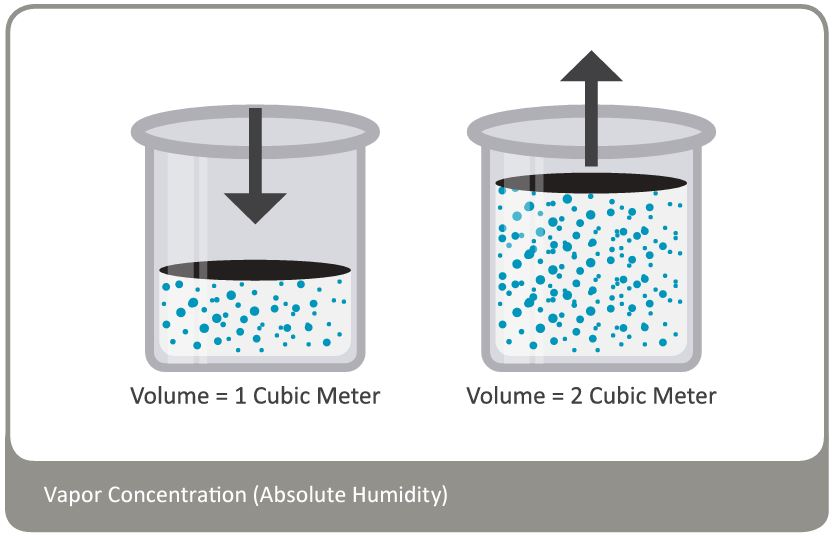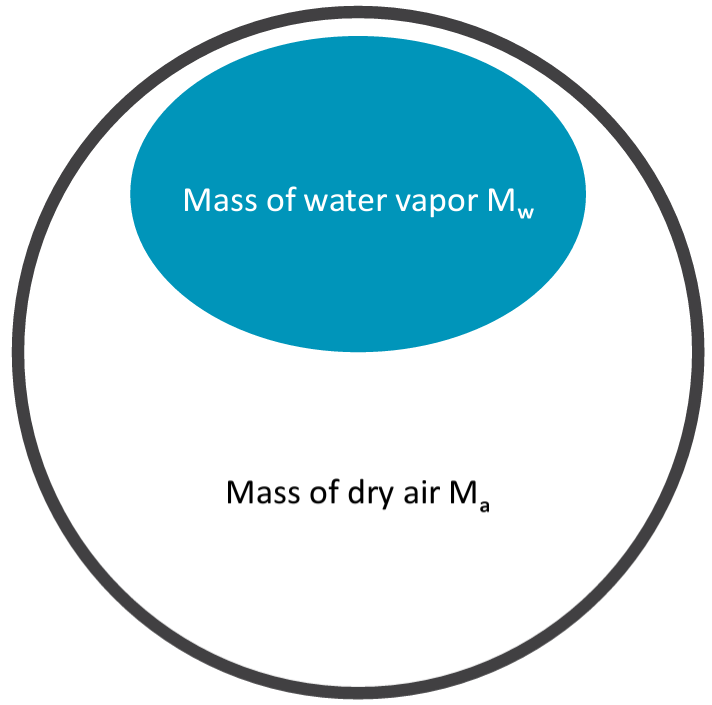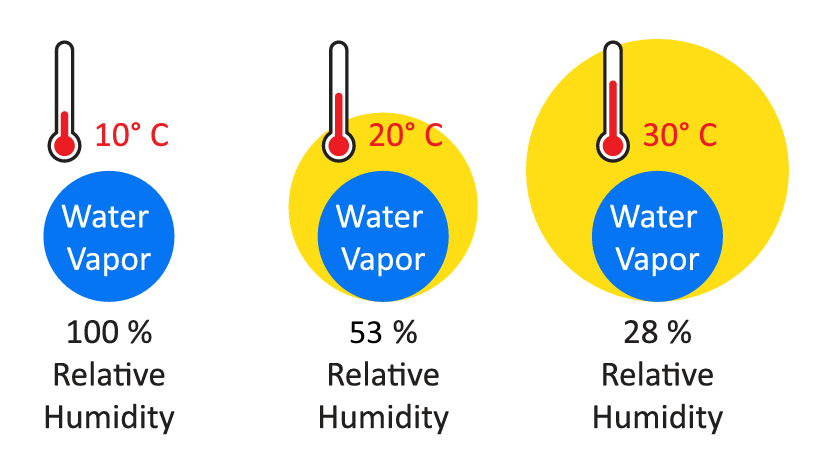﻿ Definitions of Humidity: Vapor Concentration

# Humidity Academy Theory 4 – Definitions of Humidity: Vapor Concentration

## Vapor Concentration (Absolute Humidity)

The vapor concentration or absolute humidity of a mixture of water vapor and dry air is defined as the ratio of the mass of water vapor Mw to the volume V occupied by the mixture.

Dv = Mw / V expressed in grams/m3 or in grains/cu ft

The value of Dv can be derived as follows from the equation PV = nRT

Mw = nw x mw where
nw = number of moles of water vapor present in the volume V
mw = molecular mass of water

Dv = Mw / V = nw x mv / V = mw x p / RT where
mw = 18.016 gram
p = partial pressure of water vapor [Pa]
R = 8.31436 Pa x m3 / K x mole
T = temperature of the gas mixture in KDv = p / 0.4615 x T [g / m3]
1 gr (grain) = 0.0648 g (gram)
1 cu ft = 0.0283168 m3

Dv [gr / cu ft] = 0.437 x Dv [g / m3]

## Specific Humidity

Specific humidity is the ratio of the mass Mw of water vapor to the mass (Mw + Ma) of moist air.

Q = Mw / (Mw + Ma)

Q = p mw w / (p mw + (Pb – p) maa)

Q = 1000 p / (1.6078 Pb – 0.6078 p) [g / kg]

1 gr (grain) = 0.0648 g (gram)
1 lb = 0.4535923 kg

Q [gr / lb] = 7 x Q [g / kg]

## Mixing Ratio

The mixing ratio r of moist air is the ratio of the mass Mw of water vapor to the mass Ma of dry air with which the water vapor is associated:

r = Mw / Ma

Mw = nw x Mw = Mw x p V / RT
Ma = na x ma = ma x pa V / RT = ma x (Pb – p) V / RT, where:
Nw = number of moles of water vapor present in the volume V
na = number of moles of dry air present in the volume V
Mw = 18.016 gramma = 28.966 gra
ma = partial pressure of water vapor [Pa]
p = partial pressure of water vapor [Pa]
pa = partial pressure of dry air [Pa]
Pb = total or barometric pressure [Pa]
R = 8.31436 Pa x m3 / K x mole
T = temperature of the gas mixture in K
V = volume occupied by the air – water vapor mixturer = Mw p / ma (Pb – p)

r = 621.97 x p / (Pb – p) [g / kg]

1 gr (grain) = 0.0648 g (gram)
1 lb = 0.4535923 kg

r [gr / lb] = 7 x r [g / kg]

## Volume Mixing Ratio

The volume mixing ratio is the ratio of number of moles of water vapor Nw to the number of moles of dry air na with which the water vapor is associated.

This usually expressed in terms of parts per million:
PPMv = 106 x Nw / na

Nw = p V / RT
na = pa V / RT = ma x (Pb – p) V / RT, where:
p = partial pressure of water vapor [Pa]
pa = partial pressure of dry air [Pa]
Pb = total or barometric pressure [Pa]
R = 8.31436 Pa x m3 / K x mole
T = temperature of the gas mixture in K
V = volume occupied by the air – water vapor mixture

PPMv = 106 x p / (Pb – p)

## Relative Humidity (rh)Relative humidity is the ratio of two pressures:

%rh = 100 x p/ps

where p is the actual partial pressure of the water vapor present in the ambient and ps the saturation pressure of water at the temperature of the ambient.
Relative humidity sensors are usually calibrated at normal room temperature (well above freezing). Consequently, it is generally accepted that this type of sensor indicates relative humidity with respect to water at all temperatures (including below freezing). As already noted, ice produces a lower vapor pressure than liquid water. Therefore, when ice is present, saturation occurs at a relative humidity of less than 100 %rh. For instance, a humidity reading of 75 %rh at a temperature of -30 °C, corresponds to saturation above ice.